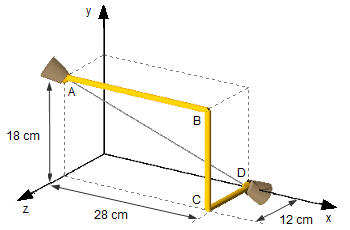Rotational Kinematics

The ABCD rod shown rotates on two spherical joints in A and D, in a clockwise direction when viewed from the point of view A. The angular speed of the bar at the instant considered is equal to 12 rad/s, and decreases at the rate of 3 rad/s2. Find:
a) The angular velocity, in rad/s;
b) The angular acceleration, in rad/s2;
c) The velocity of point B, in em m/s;
d) The acceleration of point B, in m/s2.